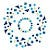# Regression in PyCaret!!!

## Let us first understand what Regression analysis is.

→ Linear regression was the first type of regression analysis to be studied rigorously and to be used extensively in practical applications.
→ Linear Regression works on building a “Linear Relationship” between the independent and dependent variables in the data.
* When only one independent variable is present then the Linear regression can be said to be “Simple Linear Regression”.
* In the case of multiple independent features, the Linear Regression can be said to be “Multiple Linear Regression”.

# Regression Using Scikit-learn

`import pandas as pdimport numpy as npfrom sklearn.model_selection import train_test_splitfrom sklearn.metrics import mean_squared_error,r2_score,mean_absolute_errorfrom catboost import CatBoostRegressorfrom sklearn.linear_model import LinearRegressionfrom sklearn.ensemble import RandomForestRegressorfrom xgboost import XGBRegressorfrom sklearn.tree import DecisionTreeRegressor`
`data = pd.read_csv('/contents/boston.csv')data.isnull().sum()`

Handling outliers is also a important task, However for the sake of simplicity I will be skipping the step.

`x = data.drop('medv',axis = 1)y = data['medv']x_train,x_test,y_train,y_test = train_test_split(x,y,test_size = 0.3,random_state = 123)`
`linear_model = LinearRegression().fit(x_train,y_train)decision_tree = DecisionTreeRegressor().fit(x_train,y_train)random_model = RandomForestRegressor().fit(x_train,y_train)xgb_model = XGBRegressor().fit(x_train,y_train)cat_model = CatBoostRegressor().fit(x_train,y_train)`
`def evaluate_Regression_models(model,x_test,y_test):  prediction = model.predict(x_test)  print("Mean Absolute Error:",                       mean_absolute_error(y_test,prediction))  print("Mean Squared Error : ",                        mean_squared_error(y_test,prediction))  print("Root Mean Squared Error : ",                     np.sqrt(mean_squared_error(y_test,prediction)))  print("R2 Score : ",r2_score(y_test,prediction))`
• Linear Regression
• Decision Tree
• RandomForest
• XGBoost
• CatBoost

If we look at all this in a single frame it comes around to 30 lines, even though hyperparameter tuning and outlier handling was not done.

`import pandas as pdimport numpy as npfrom sklearn.model_selection import train_test_splitfrom sklearn.metrics import mean_squared_error,r2_score,mean_absolute_errorfrom catboost import CatBoostRegressorfrom sklearn.linear_model import LinearRegressionfrom sklearn.ensemble import RandomForestRegressorfrom xgboost import XGBRegressorfrom sklearn.tree import DecisionTreeRegressordata = pd.read_csv('/contents/boston.csv')data.isnull().sum()x = data.drop('medv',axis = 1)y = data['medv']x_train,x_test,y_train,y_test = train_test_split(x,y,test_size = 0.3,random_state = 123)linear_model = LinearRegression().fit(x_train,y_train)decision_tree = DecisionTreeRegressor().fit(x_train,y_train)random_model = RandomForestRegressor().fit(x_train,y_train)xgb_model = XGBRegressor().fit(x_train,y_train)cat_model = CatBoostRegressor().fit(x_train,y_train)def evaluate_Regression_models(model,x_test,y_test):  prediction = model.predict(x_test)  print("Mean Absolute Error:",                       mean_absolute_error(y_test,prediction))  print("Mean Squared Error : ",                        mean_squared_error(y_test,prediction))  print("Root Mean Squared Error : ",                     np.sqrt(mean_squared_error(y_test,prediction)))  print("R2 Score : ",r2_score(y_test,prediction))`

What if I tell you all these 25 lines of code could be reduced to a mere 10 lines of code including hyperparameter tuning.

# Getting Started with Regression in PyCaret!!

If you are not familiar with PyCaret. I suggest you to first go through the below link before moving on from here.

Complete Guide to PyCaret.

`import pycaretfrom pycaret.regression import *data = pd.read_csv('/contents/boston.csv')`

We will work with the boston data.

## Setting up the PyCaret environment

`reg = setup(data = data, target = 'medv')`

After this press enter and you will get results as shown below.

## Compare models

`compare_models()`

# Creating Models

`CBR = create_model('catboost')`

Model ID for Regression Models.

`+------------+-----------------------------------+|     ID     |               Name                |+------------+-----------------------------------+| ‘lr’       | Linear Regression                 || ‘lasso’    | Lasso Regression                  || ‘ridge’    | Ridge Regression                  || ‘en’       | Elastic Net                       || ‘lar’      | Least Angle Regression            || ‘llar’     | Lasso Least Angle Regression      || ‘omp’      | Orthogonal Matching Pursuit       || ‘br’       | Bayesian Ridge                    || ‘ard’      | Automatic Relevance Determination || ‘par’      | Passive Aggressive Regressor      || ‘ransac’   | Random Sample Consensus           || ‘tr’       | TheilSen Regressor                || ‘huber’    | Huber Regressor                   || ‘kr’       | Kernel Ridge                      || ‘svm’      | Support Vector Machine            || ‘knn’      | K Neighbors Regressor             || ‘dt’       | Decision Tree                     || ‘rf’       | Random Forest                     || ‘et’       | Extra Trees Regressor             || ‘ada’      | AdaBoost Regressor                || ‘gbr’      | Gradient Boosting Regressor       || ‘mlp’      | Multi Level Perceptron            || ‘xgboost’  | Extreme Gradient Boosting         || ‘lightgbm’ | Light Gradient Boosting           || ‘catboost’ | CatBoost Regressor                |+------------+-----------------------------------+`

## Tune Model

`tuned_CBR = tune_model(CBR,n_iter = 50)`

## Plot a Model

`model = create_model('Model_name')plot_model(model)`

Plot ID for Regression Models

`+-----------------------------+-------------+|            Name             |    Plot     |+-----------------------------+-------------+| Residuals Plot              | ‘residuals’ || Prediction Error Plot       | ‘error’     || Cooks Distance Plot         | ‘cooks’     || Recursive Feature Selection | ‘rfe’       || Learning Curve              | ‘learning’  || Validation Curve            | ‘vc’        || Manifold Learning           | ‘manifold’  || Feature Importance          | ‘feature’   || Model Hyperparameter        | ‘parameter’ |+-----------------------------+-------------+`

## Interpret Model

`interpret_model(tuned_CBR)`

## Predict Model

`predict_model(tuned_CBR)`

## Finalize Model

`model = create_model('Model_name')finalize_model(model)`

## Save Models

`save_model(tuned_CBR,'final_model')`

If we look at all the code lines in a single frame, we can see how PyCaret literally reduces the time to build as well as compare models.

`import pycaretfrom pycaret.regression import *data = pd.read_csv('/contents/boston.csv')reg = setup(data = data, target = 'medv')compare_models()CBR = create_model('catboost')tuned_CBR = tune_model(CBR,n_iter = 50)interpret_model(tuned_CBR)predict_model(tuned_CBR)finalize_model(model)`

It is clear how PyCaret’s Low code approach can boost the experimentation time for data scientist and come to solution without wasting time on codes.

# Visit us on https://www.insaid.co/